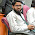Inductance of Three Phase Lines With Equilateral and Symmetrical Spacing

Consider a three phase line consisting of three phase conductors a, b and c as shown in the Fig. 1. These three conductors are equally spaced at the corners of an equilateral triangle having radius r.Fig. 1

The flux linkages of conductor a are given by,
If the currents are assumed to be balanced than
Ia+ Ib + Ic = 0
...                Ia = - ( Ib + Ic )
or               ( Ib + Ic) = - Ia
The above equation becomes,
The inductance of conductor a is given by
because of symmetry, conductors b and c will have same inductance as that of conductor a. Each phase consists of only one conductor. So the above equation gives inductance per phase of the three phase lines. For stranded conductor we write Ds whereas for single conductor it is replaced by r'.
1.